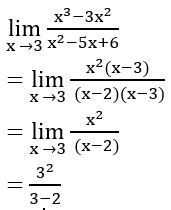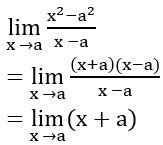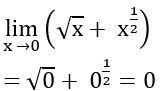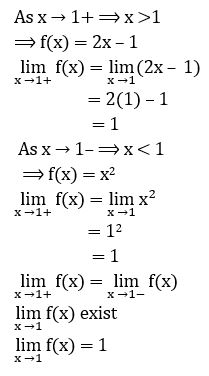Welcome To Basics In Maths

# Limits (Q’s & Ans) || V.S.A.Q’S||Limits (Q’s & Ans) || V.S.A.Q’S|| designed by the ‘Basics in Maths‘ team. These notes to do help the intermediate First-year Maths students.

Inter Maths – 1B two marks questions and solutions are very useful in IPE examinations.

## Limits  V.S.A.Q’S

Limits

Question 1

Sol:= 9

Question 2

Sol:= a + a = 2a

Question 3

Sol:Question 4

Sol:

we know that if x > 0

As x → 0+ ⟹ x > 0

As x → 0+ ⟹ x < 0

Question 5

Sol:1/5